# Search

About 15 Search Results Matching Types of Worksheet, Worksheet Section, Generator, Generator Section, Type matching Worksheet Section, Grades matching 4th Grade, Similar to Valentine's Day Addition Worksheet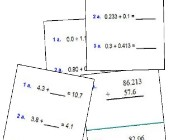## Adding Decimals Worksheets

Help kids practice decimal addition with the follo...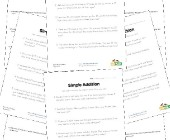## Word Problem Worksheets

Help children develop their problem solving skills...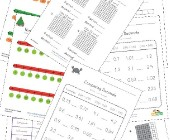## Decimal Worksheets

This collection of free decimal worksheets will he...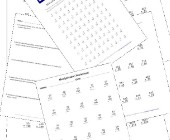## Multiplication Worksheets for Kids

This set of math worksheets is geared towards help...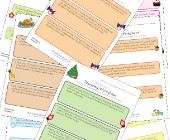## Rounding Worksheets

Check out our collection of rounding worksheets in...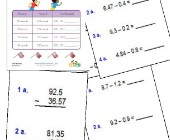## Subtracting Decimals Worksheets

These worksheets will help children practice decim...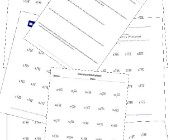## Division Worksheets

Help kids learn division with this collection of m...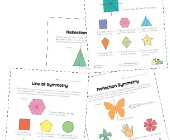## Symmetry Worksheets

Help kids learn about the concept of symmetry with...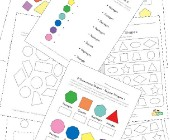## Geometry Worksheets

Help kids learn the early basics of geometry inclu...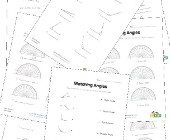## Angles Worksheets

Help kids learn about angles with this collection ...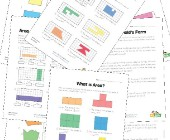## Area and Perimeter Worksheets

Help kids learn to calculate the area and perimete...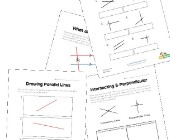## Parallel, Perpendicular and Intersecting Lines Worksheets

Help kids learn about lines including parallel lin...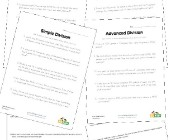## Division Word Problems

Practice division and problem solving with these d...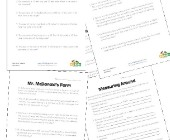## Area and Perimeter Word Problems

This collection of area and perimeter worksheets c...